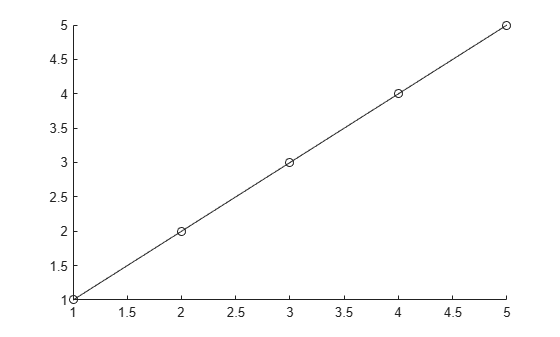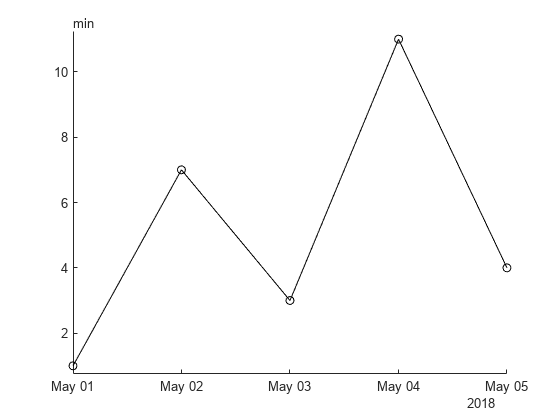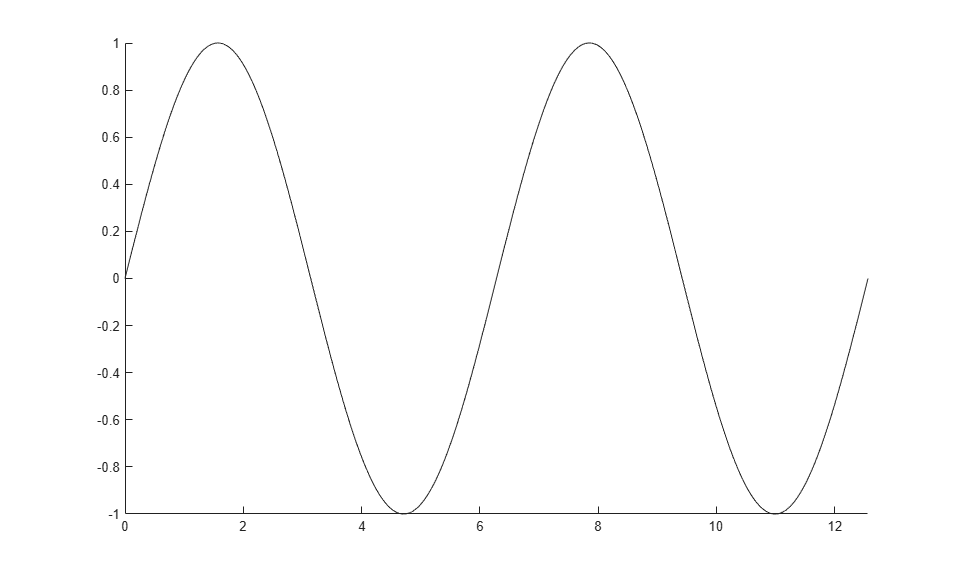## Syntax

``addpoints(an,x,y)``
``addpoints(an,x,y,z)``

## Description

example

````addpoints(an,x,y)` adds points defined by `x` and `y` to the animated line specified by `an`. Create an animated line with the `animatedline` function. To display the updates on the screen, use `drawnow` or ```drawnow limitrate```. New points automatically connect to previous points. ```
````addpoints(an,x,y,z)` adds points defined by `x`, `y`, and `z` to the 3-D animated line specified by `an`.```

## Examples

collapse all

Create an animated line object with no data. Then, add five points to the line. Use a circle to mark each point.

```h = animatedline('Marker','o'); x = 1:5; y = 1:5; addpoints(h,x,y)```To plot nonnumeric points, such as datetime and duration values, start by initializing the animated line with values of the type you want to plot. You can specify either the first point in your plot or placeholder values such as `NaT` or `NaN`.

For example, plot datetime values on the x-axis and duration values (minutes) on the y-axis. Initialize the animated line with a `NaT` value and a `minutes(NaN)` value. Then create a datetime vector (`x`) and a duration vector (`y`) and add the points in those vectors to the animated line.

```an = animatedline(NaT,minutes(NaN),"Marker","o"); x = datetime(2018,5,1:5); y = minutes([1 7 3 11 4]); addpoints(an,x,y)```Create an animated line using the `animatedline` function. Then, add points to the line within a loop to create an animation. Set the axis limits before the loop to prevent the limits from changing.

```figure h = animatedline; axis([0 4*pi -1 1]) for x = linspace(0,4*pi,10000) y = sin(x); addpoints(h,x,y) drawnow limitrate end ```## Input Arguments

collapse all

`AnimatedLine` object. Create an `AnimatedLine` object using the `animatedline` function.

First coordinate values, specified as a scalar or a vector. For Cartesian axes, the first coordinate is x-axis position. For geographic axes, the first coordinate is latitude in degrees.

The length of `x` must equal the length of `y`.

Example: `11:20`

Data Types: `single` | `double` | `int8` | `int16` | `int32` | `int64` | `uint8` | `uint16` | `uint32` | `uint64` | `datetime` | `duration`

Second coordinate values, specified as a scalar or a vector. For Cartesian axes, the second coordinate is y-axis position. For geographic axes, the second coordinate is longitude in degrees.

The length of `y` must equal the length of `x`.

Example: `11:20`

Data Types: `single` | `double` | `int8` | `int16` | `int32` | `int64` | `uint8` | `uint16` | `uint32` | `uint64` | `datetime` | `duration`

Third coordinate values, specified as a scalar or a vector. For Cartesian axes, the third coordinate is z-axis position.

The length of `z` must equal the length of `x` and `y`.

Example: `11:20`

Data Types: `single` | `double` | `int8` | `int16` | `int32` | `int64` | `uint8` | `uint16` | `uint32` | `uint64` | `datetime` | `duration`

## Version History

Introduced in R2014b

expand all